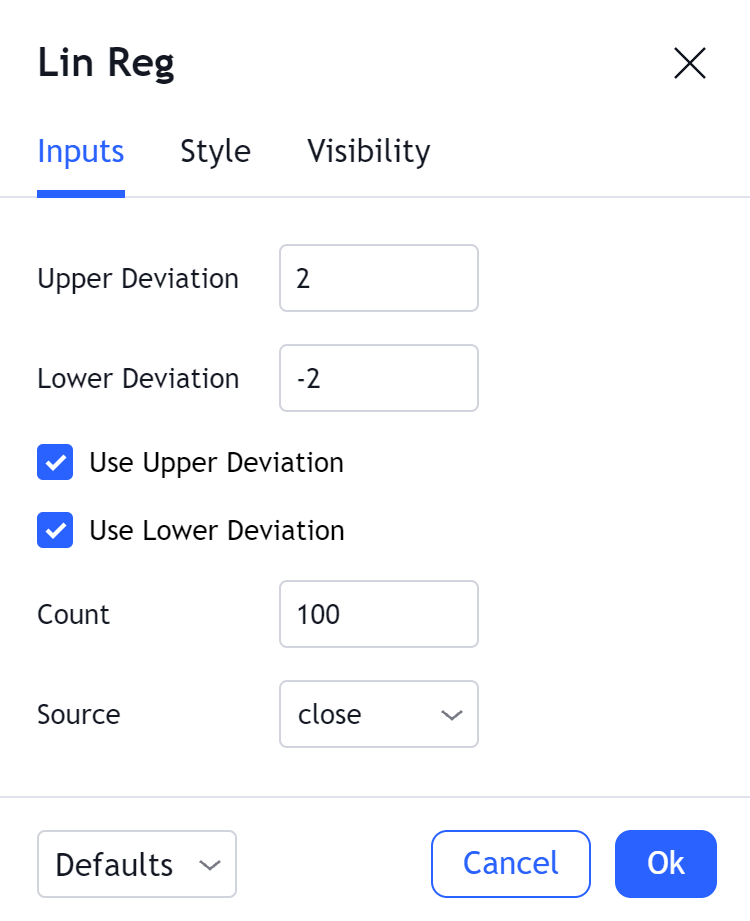# Linear Regression

#### Definition

The Linear Regression indicator visualizes the general price trend of a specific part of the chart based on the Linear Regression calculation. The central line of the indicator is the linear regression calculation itself, and the lines above or below it are X number of standard deviations removed from the main one. This is a good tool to use to determine when a price is unusually far away from its baseline.

The bands indicate support and resistance; when the prices stay outside the channel for a significant period of time, the reversal might be expected ahead.

#### Inputs##### Upper Deviation

Determines the number of standard deviations away from the base to set the upper channel. Essentially this sets the distance between the center base and the edge of the upper channel.

##### Lower Deviation

Determines the number of standard deviations away from the base to set the lower channel. Essentially this sets the distance between the center base and the edge of the lower channel.

##### Use Upper Deviation

Toggles the use/visibility of the upper channel.

##### Use Lower Deviation

Toggles the use/visibility of the lower channel.

##### Count

The number of bars linear regression uses for its calculations: i.e. if the number is 100, the channel will be calculated on the last 100 bars.

##### Source

Sets the price source to calculate the channel position.